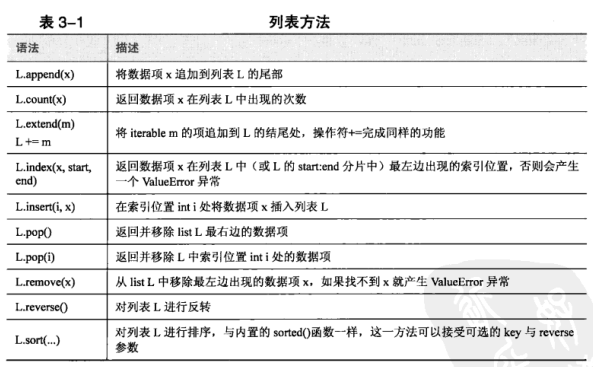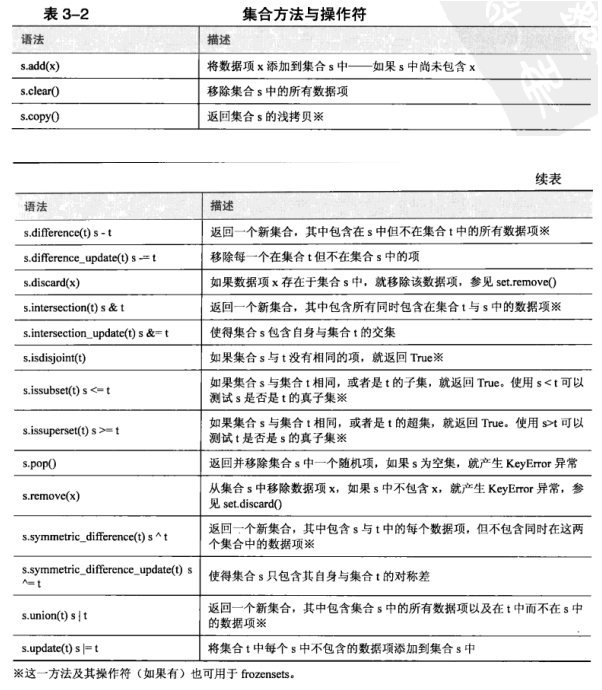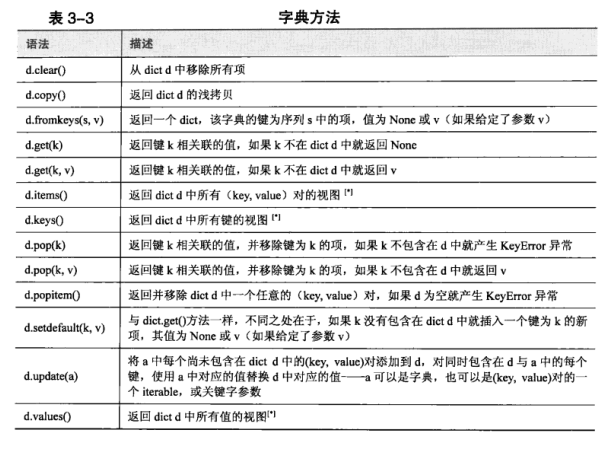# 1.序列类型

## 1.1.元组

##### tuple数据类型可以作为一个函数进行调用
• tuple()：不指定参数是返回一个空元组
• tuple(tp)：tp为元组时返回该参数的浅拷贝
• tuple(notp)：notp不为元组，尝试把notp转为元组
• ()：使用空圆括号创建一个空元组
• (1,)：包含一个或多个项的元组
• 1,2 ：通过逗号分隔，不是使用圆括号也可以创建元组
##### 元组可以使用标准的比较操作符(<，<=，==，!=，>=，>)进行比较，这种比较实际是逐项进行的（对嵌套项，比如元组内的元组，递归进行处理）
``````hair = 'black','brown','blonde','red'
hair[:2],'gray',hair[2:]
``````

(('black', 'brown'), 'gray', ('blonde', 'red'))

``````hair[:2]+('gray',)+hair[2:]
``````

('black', 'brown', 'gray', 'blonde', 'red')

##### 分片操作符[]可以应用于一个分片，必要的时候可以使用多个：
``````eyes=('brown','hazel','amber','green','blue','gray')
colors=(hair,eyes)
``````
``````colors
``````

(('black', 'brown', 'blonde', 'red'), ('brown', 'hazel', 'amber', 'green', 'blue', 'gray'))

``````colors[3:-1]
``````

('green', 'blue')

##### 元组可以存放任意数据类型的任意项，包括组合类型，比如元组与列表，实际上存放的是对象引用。但是这种复杂的嵌套数据结构很容易混淆，可以使用特定的索引位置处理。
``````MANUFACTURER,MODEL,SEATING=(0,1,2)
MINIMUN,MANNUM=(0,1)
aircraft=('Airbus','A320-200',(100,200))
aircraft[SEATING][MANNUM]
``````

200

##### 在赋值操作的右边是序列，左边是元组的情况下，我们称右边被拆分
``````a,b=1,2
a,b=(b,a)
a,b
``````

(2, 1)

## 1.2.命名的元组

• 参数1--要创建的自定义元组数据类型的名
• 参数2--一个字符串，包含使用空格分隔的名称，每个名称代表元组数据类型的一项
``````import collections
Sale=collections.namedtuple('Sale','productid customerid date quantity price')
sales=[]
sales.append(Sale(432,921,'2018-01-02',3,7.99))
sales.append(Sale(419,874,'2018-02-02',1,18.49))
sales
``````

[Sale(productid=432, customerid=921, date='2018-01-02', quantity=3, price=7.99),Sale(productid=419, customerid=874, date='2018-02-02', quantity=1, price=18.49)]

``````total=0
for sale in sales:
total+=sale.quantity*sale.price
print('Total \${0:.2f}'.format(total))
``````

Total \$42.46

## 1.3.列表

##### list数据类型可以作为函数进行调用
• list()：不带参数进行调用时将返回一个空列表
• list(lt)：lt为列表时，返回lt的浅拷贝
• list(nolt)：nolt为非列表时，尝试将nolt转为列表
• [ ]：使用空的方括号来创建空列表
• [1,2]：创建一个或多个项的列表，逗号分隔
##### 内置len()以及del语句##### 任意可迭代的（列表，元组等）数据类型都可以使用序列拆分操作符进行拆分，即*
``````first,*rest=[9,2,-4,8,7]
first,rest
``````

(9, [2, -4, 8, 7])

##### 列表可以使用内置的reversed()函数与sorted()函数进行反转和排序，列表同样包含邓建的方法：list.reverse()和list.sort()。
``````x=[1,2,3]
# 内置函数不是返回列表，而是返回一个迭代器，因此需要使用list转为列表
list(reversed(x))
``````

[3, 2, 1]

``````x=[1,2,3]
x.reverse()
x
``````

[3, 2, 1]

``````x=[4,6,2,1,7,9]
# sort()方法不返回值，实际是将x进行了修改
y=x.sort()
print('x=',x)
print('y=',y)
``````

x= [1, 2, 4, 6, 7, 9] y= None

``````x=[4,6,2,1,7,9]
# x的考虑
y=x[:]
y.sort()
print('x=',x)
print('y=',y)
``````

x= [4, 6, 2, 1, 7, 9] y= [1, 2, 4, 6, 7, 9]

``````x=[4,6,2,1,7,9]
# 使用内置函数
y=sorted(x)
print('x=',x)
print('y=',y)
``````

x= [4, 6, 2, 1, 7, 9] y= [1, 2, 4, 6, 7, 9]

#### 1.3.1.高级排序

##### sort()方法语法：list.sort(cmp=None, key=None, reverse=False)
• cmp -- 可选参数, 如果指定了该参数会使用该参数的方法进行排序。
• key -- 主要是用来进行比较的元素，只有一个参数，具体的函数的参数就是取自于可迭代对象中，指定可迭代对象中的一个元素来进行排序。
• reverse -- 排序规则，reverse = True 降序， reverse = False 升序（默认）。
``````# 获取列表的第二个元素
def takeSecond(elem):
return elem
# 列表
random = [(2, 2), (3, 4), (4, 1), (1, 3)]
# 指定第二个元素排序
random.sort(key=takeSecond)
# 输出类别
print('排序列表：', random)
``````

``````# 指定第二个元素排序
random.sort(key=takeSecond,reverse=True)
# 输出类别
print('排序列表：', random)
``````

## 1.4.列表推导式

##### 列表推导式的语法如下：
• [expression for item in iterable]
• [expression for item in iterable if condition]
##### 实际等价于
``````temp=[]
for item in iterable:
if condition:
temp.append(expression)
codes =[]
for sex in 'MF':
for size in 'SMLX':
if sex =='F' and size == 'X':
continue
for color in 'BGW':
codes.append(sex+size+color)
print(codes)
``````

['MSB', 'MSG', 'MSW', 'MMB', 'MMG', 'MMW', 'MLB', 'MLG', 'MLW', 'MXB', 'MXG', 'MXW', 'FSB', 'FSG', 'FSW', 'FMB', 'FMG', 'FMW', 'FLB', 'FLG', 'FLW']

``````codes=[sex+size+color for sex in 'MF' for size in 'SMLX' for color in 'BGW'
if not (sex =='F' and size =='X')]
print(codes)
``````

['MSB', 'MSG', 'MSW', 'MMB', 'MMG', 'MMW', 'MLB', 'MLG', 'MLW', 'MXB', 'MXG', 'MXW', 'FSB', 'FSG', 'FSW', 'FMB', 'FMG', 'FMW', 'FLB', 'FLG', 'FLW']

# 2.集合类型

set也是一种组合数据类型，支持成员关系操作符(in)，对象大小计算操作符(len())，并且也是iterable。进行迭代时，集合类型以任意顺序提供数据项。

• 所有内置的固定数据类型（eg：float,frozenset,int,str,tuple）都是可哈希运算的，都可以添加到集合中。内置的可变数据类型（eg:dict,list,set）都是不可哈希的，其哈希值会随着包含项数的变化而变化，因此，这些数据类型不能添加到集合中。
``````s=set()
``````

TypeError Traceback (most recent call last)
TypeError: unhashable type: 'list'

• 集合类型可以使用标准的比较操作符（<,<=,==,!=,>=,>）进行比较，要注意的是，操作符==与!=都使用的是其通常的含义，其比较的方式是逐项比较（对嵌套项，比如集合内的元组或固定集合，则递归比较），其他比较操作符则进行子集比较火超集比较。

## 2.1.集合

##### set数据类型可以作为函数进行调用
• set()---不带参数进行调用时将返回一个空集合
• set(st)---st为set类型，则返回st的浅拷贝
• set(nost)---nost为非set类型，则将尝试将给定的对象转换为集合。
• {1,2,3,4,5}---使用花括号，通过逗号分隔
##### 集合中包含的每个数据项都是独一无二的---添加重复的数据项固然不会引发问题，但是会覆盖。
``````# 删除重复项
x = [1,1,2,3,4,5,5]
x = list(set(x))
print(x)
``````

[1, 2, 3, 4, 5]

##### 集合支持内置的len()函数，也支持使用in与not in进行的快速成员关系测试。##### 集合还提供了常用的集合操作符
``````s=set('pecan')
t=set('pie')
print('s|t:',s|t)
print('s.union(t):',s.union(t))
``````

s|t: {'e', 'i', 'a', 'c', 'n', 'p'} s.union(t): {'e', 'i', 'a', 'c', 'n', 'p'}

``````s=set('pecan')
t=set('pie')
print('s&t:',s&t)
print('s.intersection(t):',s.intersection(t))
``````

s&t: {'e', 'p'} s.intersection(t): {'e', 'p'}

##### 集合也可用于删除不需要的数据项
``````s=('a','b','c','d','e')
f={'b','c'}
print(set(s)-f)
``````

{'d', 'a', 'e'}

## 2.2.集合推导式

##### 集合推导式的语法类似于列表生成，如下
• {expression for item in iterable}
• {expression for item in iterable if condition}
``````s={x for x in range(10) if x%2==0}
print(type(s),'=',s)
``````

<class 'set'> = {0, 2, 4, 6, 8}

## 2.3.固定集合

##### 固定集合是指那种一旦创建就不能改变的集合。固定集合只能使用frozenset数据类型函数进行创建。
• frozenset()---不带参数，将返回一个空的固定集合
• frozenset(fst)---fst为固定集合，则返回该参数的浅拷贝
• frozenset(nofst)---nofst为非固定集合，则尝试将给定的对象转换为frozenset

# 3.映射类型

• 3.0支持两种无序的映射类型--内置的dict类型以及标准库中的collections.defaultdict类型。3.1中引入了新的有序映射类型colletctions.OrderedDict
• 只有可哈希运算的对象可用作字典的键，因此，固定的数据类型（float,frozenset,int,str以及tuple）都可以用作字典的键。每个键对应的值实际上是对象引用，可以引用任意类型的对象，包括数字，字符串，列表，集合，字典，函数等。
• 字典类型可以使用标准的比较操作符（<,<=,==,!=,>=,>）进行比较，这种比较实际是逐项进行的（对嵌套项，比如字典内的元组或字典，递归进行处理）。对于字典而言，有意义的比较操作符是==和!=。

## 3.1.字典

dict是一种无序的组合数据类型，其中包含0个或多个键值对。其中，键是指向可哈希运算的对象的对象引用，值时可以指向任意类型对象的对象引用。字典是可变的，且无序的。

##### dict数据类型可以作为函数调用
• dict()---不带参数，返回一个空字典
• dict(dt)---dt是映射类型，则返回该参数为基础的字段。
• dict([('a','1'),('b','2')])---使用序列尾参数是，序列中每个数据项必须包括2个对象，其中一个为键，一个为值。
• {}---空字典
``````d1 = dict({'id':1948,'name':'Washer','size':3})
print(d1)
``````

{'id': 1948, 'name': 'Washer', 'size': 3}

``````d2 = dict(id=1948,name='Washer',size=3)
print(d2)
``````

{'id': 1948, 'name': 'Washer', 'size': 3}

``````d3 = dict([('id',1948),('name','Washer'),('size',3)])
print(d3)
``````

{'id': 1948, 'name': 'Washer', 'size': 3}

``````d4=dict(zip(('id','name','size'),(1948,'Washer',3)))
print(d4)
``````

{'id': 1948, 'name': 'Washer', 'size': 3}

``````d5={'id':1948,'name':'Washer','size':3}
print(d5)
``````

{'id': 1948, 'name': 'Washer', 'size': 3}

``````d6={}.fromkeys('ABCD',3)
print(d6)
``````

{'A': 3, 'B': 3, 'C': 3, 'D': 3}

##### 方括号可以用于添加或删除字典项，要添加一个项，可以使用操作符=；要删除一个项，可以使用del语句。##### 迭代方法
``````for key,value in d1.items():
print(key,'=',value)
``````

id = 1948 name = Washer size = 3

``````# 方法iteritems在3.x中被废除
for key,value in d1.iteritems():
print(key,'=',value)
``````
``````---------------------------------------------------------------------------
AttributeError                            Traceback (most recent call last)
<ipython-input-28-68e0b9e35b7f> in <module>
1 # 方法iteritems在3.x中被废除
----> 2 for key,value in d1.iteritems():
3     print(key,'=',value)

AttributeError: 'dict' object has no attribute 'iteritems'
``````
``````for key in d1.keys():
print(key,'=')
``````

id =
name =
size =

``````for value in d1.values():
print('=',value)
``````

= 1948 = Washer
= 3

``````for key in d1:
print(key,'=',d1[key])
``````

id = 1948
name = Washer
size = 3

##### 成员关系操作符in用来查询某个特定的键是否存在于字典中。也可以使用联合操作符来查看来自给定集合的那些键存在于字典中。
``````print('id' in d1)
``````

True

``````s={'id','size','length'}
matches=d1.keys() & s
print(matches)
``````

{'id', 'size'}

## 3.2.字典推导式

• {keyexpression:valueexpression for key,value in iterable}
• {keyexpression:valueexpression for key,value in iterable if condition}
##### 字典推导式也可以用来反转
``````inverted = {v:k for k,v in d1.items()}
print(inverted)
``````

{1948: 'id', 'Washer': 'name', 3: 'size'}

## 3.3.默认字典

• default_factory---指定key值不存在时，默认值的取值函数
##### 语法：
• defaultdict() --空字典

#### 参考链接

Python——collections模块

``````import collections
dd1=collections.defaultdict()
print(dd1)
``````

defaultdict(None, {})

``````import collections
dd2=collections.defaultdict(int)
print(dd2)
dd2=('a')
dd2=('b')
print(dd2)
``````

defaultdict(<class 'int'>, {})
defaultdict(<class 'int'>, {1: 'a', 2: 'b'})

``````print(d1['length'])
``````
``````---------------------------------------------------------------------------
KeyError                                  Traceback (most recent call last)
<ipython-input-37-45fa0cc9c216> in <module>
----> 1 print(d1['length'])

KeyError: 'length'
``````
``````print(dd2['length'])
print(dd2)
``````

0
defaultdict(<class 'int'>, {1: 'a', 2: 'b', 'length': 0})

``````import collections
dd3 = collections.defaultdict(lambda: 'defaultValue')
print('dd3=',dd3)
dd3='aaa'
dd3['1']='bbb'
print('dd3=',dd3)
print("dd3['length']=",dd3['length'])
print('dd3=',dd3)
``````

dd3= defaultdict(`<function <lambda> at 0x000001FFB4E02268>`, {})
dd3= defaultdict(`<function <lambda> at 0x000001FFB4E02268>`, {0: 'aaa', '1': 'bbb'})
dd3['length']= defaultValue
dd3= defaultdict(`<function <lambda> at 0x000001FFB4E02268>`, {0: 'aaa', '1': 'bbb', 'length': 'defaultValue'})

## 3.4.有序字典

• 注意：有序字典在创建时如果接收了无序的dict或关键字参数，则数据项顺序是任意的。这是因为，Python在底层会使用无序的dict传递关键字参数。使用update()方法也有类似的情况。故而，最好避免在创建有序字典时传递关键字参数或无序dict，也最好不要使用update()方法。
``````import collections
od1 = collections.OrderedDict([('a',1),('c',3),('b',2)])
print(od1)
``````

OrderedDict([('a', 1), ('c', 3), ('b', 2)]

``````od2 = collections.OrderedDict()
od2['a']=12
od2['z']=15
od2['c']=14
print(od2)
``````

OrderedDict([('a', 12), ('z', 15), ('c', 14)])

• 有序字段另一个特性就是，如果改变某个数据项（已经存在）的值，则顺序不会发生变化。但如果数据项不存在，则认为是新增，顺序为最后。
``````od1['c']=4
print('od1=',od1)
``````

od1= OrderedDict([('a', 1), ('c', 4), ('b', 2)])

``````od1['d']=5
print('od1=',od1)
``````

od1= OrderedDict([('a', 1), ('c', 4), ('b', 2), ('d', 5)])

• 如果想要将某个数据项移动到尾部，就必须删除该数据项，之后再重新插入
• 有序字典另一种用途是生成排序字典。
``````d = collections.OrderedDict(sorted(od1.items()))
print(d)
``````

OrderedDict([('a', 1), ('b', 2), ('c', 4), ('d', 5)])

# 4.迭代

Python中关于迭代有三个概念，第一个是Iterable，第二个是Iterator，第三个是Generator。下面就详细讲述。

##### 首先，可直接作用于for循环的数据类型：
1. 可迭代对象，如list、tuple、dict、set、str等集合类型和实现`__iter__`的可迭代对象。
2. 迭代器，如实现了`__iter__``__next__`方法的可迭代对象。
3. 生成器，如通过yield实现的function
4. 在类中实现了如果只实现`__getitem__()`的对象可以通过`iter()`函数转化成迭代器但其本身不是可迭代对象。所以当一个对象能够在for循环中运行，但不一定是Iterable对象。
5. 文件对象
##### 一个对象能够在for循环中运行，但不一定是Iterable对象。

list、dict、str虽然是可迭代的，却不是迭代器:这是因为Python的Iterator对象表示的是一个数据流，Iterator对象可以被next()函数调用并不断返回下一个数据，直到没有数据时抛出StopIteration错误。可以把这个数据流看做是一个有序序列，但我们却不能提前知道序列的长度，只能不断通过next()函数实现按需计算下一个数据，所以Iterator的计算是惰性的，只有在需要返回下一个数据时它才会计算。Iterator甚至可以表示一个无限大的数据流，例如全体自然数。而使用list是永远不可能存储全体自然数的。

##### 三者包含关系如下：

`iterable->iterator->generator`

##### 参考链接

Python迭代器,生成器(iterator,generator)详解

## 4.1.Iterable

• 示例1，可迭代对象：
``````from collections import Iterator,Iterable,Generator
# 非迭代器,只是可以由next调用的对象
class MyObject(object):
def __init__(self):
self.data=1

def __next__(self):
if self.data > 5:
raise StopIteration
else:
self.data+=1
return self.data

# 可迭代对象
class MyIterable(object):
def __init__(self):
self.mydata = MyObject()

def __iter__(self):
return self.mydata

myl = MyIterable()
myo = MyObject()
for num in myl:
print(num)

print("MyIterable "+(' is ' if isinstance(myl,Iterable) else ' is not ')+" Iterable")
print("MyObject "+(' is ' if isinstance(myo,Iterator) else ' is not ')+" Iterator")
print("MyIterable "+(' is ' if isinstance(myl,Generator) else ' is not ')+" Generator")

print(next(myo))
``````
``````2
3
4
5
6
MyIterable  is  Iterable
MyObject  is not  Iterator
MyIterable  is not  Generator
2
``````
##### 由上述示例可知：
• 可迭代对象仅含有`__iter__`的内部方法，你可以通过封装`next()`方法（python3中为`__next__()`）来将其做成一个迭代器，以生成器（generator，特殊的函数类型的迭代器）为例，你可以通过yield关键字来做一个迭代器，只不过名字被叫做generator，yield可以看做就是为对象添加了`__iter__`方法和指示下一次迭代的`next()/__next__()`方法。
• 使用`isinstance(实体名,Iterable)`可判断是否为可迭代对象
##### 示例2，非可迭代对象
``````from collections import Iterator,Iterable,Generator

class MyObject(object):

def __init__(self):
self.data=[1,2,3,4,5,6]

def __getitem__(self,i):
return self.data[i]

myo =  MyObject()
for x in iter(myo):
print(x)

print("MyObject "+(' is ' if isinstance(myo,Iterable) else ' is not ')+" Iterable")
print("MyObject "+(' is ' if isinstance(myo,Iterator) else ' is not ')+" Iterator")
print("iter(myo) "+(' is ' if isinstance(iter(myo),Iterator) else ' is not ')+" Iterator")
``````

1
2
3
4
5
6
MyObject is not Iterable
MyObject is not Iterator
iter(myo) is Iterator

## 4.2.Iterator

``````from collections import Iterator,Iterable,Generator
# 迭代器
class MyIterator(object):
def __init__(self):
self.data = 1

def __iter__(self):
return self

def __next__(self):
if self.data > 5:
raise StopIteration
else:
self.data+=1
return self.data

myiter = MyIterator()
for num in myiter:
print(num)

print("MyIterator "+(' is ' if isinstance(myiter,Iterable) else ' is not ')+" Iterable")
print("MyIterator "+(' is ' if isinstance(myiter,Iterator) else ' is not ')+" Iterator")
``````

2
3
4
5
6
MyIterator is Iterable
MyIterator is Iterator

##### 由上述示例可知：
• iterator必定是iterable的，因此其必然含有`__iter__`方法，此方法保证了iterator是可以迭代的，可以将`__iter__()`方法看做迭代器的入口，此入口告诉python对象是可for循环的，如果还为class定义了`__next__`方法时for循环会直接调用`__next__()`方法进行迭代，因此对于实现了`__next__`方法的迭代器来讲`__iter__`方法是一个不可或缺的方法，一般都返回迭代器本身（如上述示例中的self）。
• 使用isinstance(实体名,Iterator)可判断是否为迭代器

## 4.3.Generator

##### Python有两种实现生成器的方式：
1. 生成器函数：还是使用 def 定义函数，但是，使用yield而不是return语句返回结果。yield语句一次返回一个结果，在每个结果中间，挂起函数的状态，以便下次从它离开的地方继续执行。
2. 生成器表达式：类似于列表推导，只不过是把一对大括号[]变换为一对小括号()。但是，生成器表达式是按需产生一个生成器结果对象，要想拿到每一个元素，就需要循环遍历。
##### 示例1，生成器表达式
``````print([x for x in range(5)])
print(type([x for x in range(5)]))
print('-'*20)
print(next(x for x in range(5)))
print(type((x for x in range(5))))
``````

## ` [0, 1, 2, 3, 4]``<class 'list'>`

0
`<class 'generator'>`

##### 示例2，yield函数
``````def triangles():
L = 
while True:
yield L
L.append(0)
# 当i=0时，L[-1]代表列表最后一个元素(善于利用python切片特性！)
L = [L[i - 1] + L[i] for i in range(len(L))]

n = 0
for t in triangles():
print(t)
n = n + 1
if n == 10:
break

print('-'*20)
print(type(triangles))
print(type(triangles()))
``````
``````
[1, 1]
[1, 2, 1]
[1, 3, 3, 1]
[1, 4, 6, 4, 1]
[1, 5, 10, 10, 5, 1]
[1, 6, 15, 20, 15, 6, 1]
[1, 7, 21, 35, 35, 21, 7, 1]
[1, 8, 28, 56, 70, 56, 28, 8, 1]
[1, 9, 36, 84, 126, 126, 84, 36, 9, 1]
--------------------
<class 'function'>
<class 'generator'>
``````

### 示例3，`send`和`__next__`方法

#### send和__next__()区别:

1. send和next()都是让生成器向下走⼀次
2. send可以给上⼀个yield的位置传递值, 不能给后⼀个yield发送值。在第⼀次执行生成器代码的时候不能使用send()
``````def eat():
print("我吃什么啊")
a =  yield  "馒头"
print("a=",a)
b =  yield  "大饼"
print("b=",b)
c =  yield  "韭菜盒子"
print("c=",c)
yield  "GAME OVER"

gen = eat()      # 获取⽣成器

ret1 = gen.__next__()
print('__next__')
print(ret1)
print('ret1')
ret2 = gen.send("胡辣汤")
print(ret2)
print("ret2")
ret3 = gen.send("狗粮")
print(ret3)
ret4 = gen.send("猫粮")
print(ret4)
``````
``````我吃什么啊
__next__

ret1
a= 胡辣汤

ret2
b= 狗粮

c= 猫粮
GAME OVER
``````

## 4.4.内建方法

### 4.4.2.next()

``````iterator -- 可迭代对象
default -- 可选，用于设置在没有下一个元素时返回该默认值，如果不设置，又没有下一个元素则会触发 StopIteration 异常。
``````### 评论(0)

ApacheCN 人工智能知识树 v1.0

Special Sponsors 贡献者：飞龙 版本：v1.0 最近总是有人问我，把 ApacheCN 这些资料看完一遍要用多长时间，如果你一本书一本书看的话，的确要用很长时间。但我觉得这是非常麻烦的，因为每本...

ApacheCN_飞龙
2019/05/18
0
0
Python编程实践 学习笔记

Practical Programming-An Introduction to Computer Science Using Python 这本书是Jennifer Campbell等著，唐学韬(汕头大学)译，全书300页，虽内容不多，但个人认为译者比较负责，如未统一...

Honghe
2013/01/04
1.8K
1

2019/11/20
0
0
Think Python 第二版每章讲解(12)[竖屏视频] - 知乎

Python学习之路
2019/12/13
0
0
python核心编程-第四章-个人笔记

1.所有的python对象都拥有三个特性： ①身份：每个对象都有唯一的身份标识自己，可用内建函数id()来得到。基本不会用到，不用太关心 >>> a = 2>>> id(2)39411616L>>> id(a)39411616L 这个例子...

2015/11/18
50
0

OSChina 周三乱弹 —— 提高不了工作效率和脸有关系

Osc乱弹歌单（2020）请戳（这里） 【今日歌曲】 @薛定谔的兄弟 ：分享洛神有语创建的歌单「我喜欢的音乐」: 1 《夏令时记录(piano.ver)》- ゆめこ 手机党少年们想听歌，请使劲儿戳（这里） ...

67
2
List的一波操作

public static void main(String[] args) { List<Entity> list = new ArrayList<>(); list.add(new Entity(1)); list.add(new Entity(2)); list.add(new Entity(3)); ......

75
0
Spring基础

30
0

hi懒喵

53
0
《C语言》—— 数组

84
0